# 高质量实时渲染：实时环境光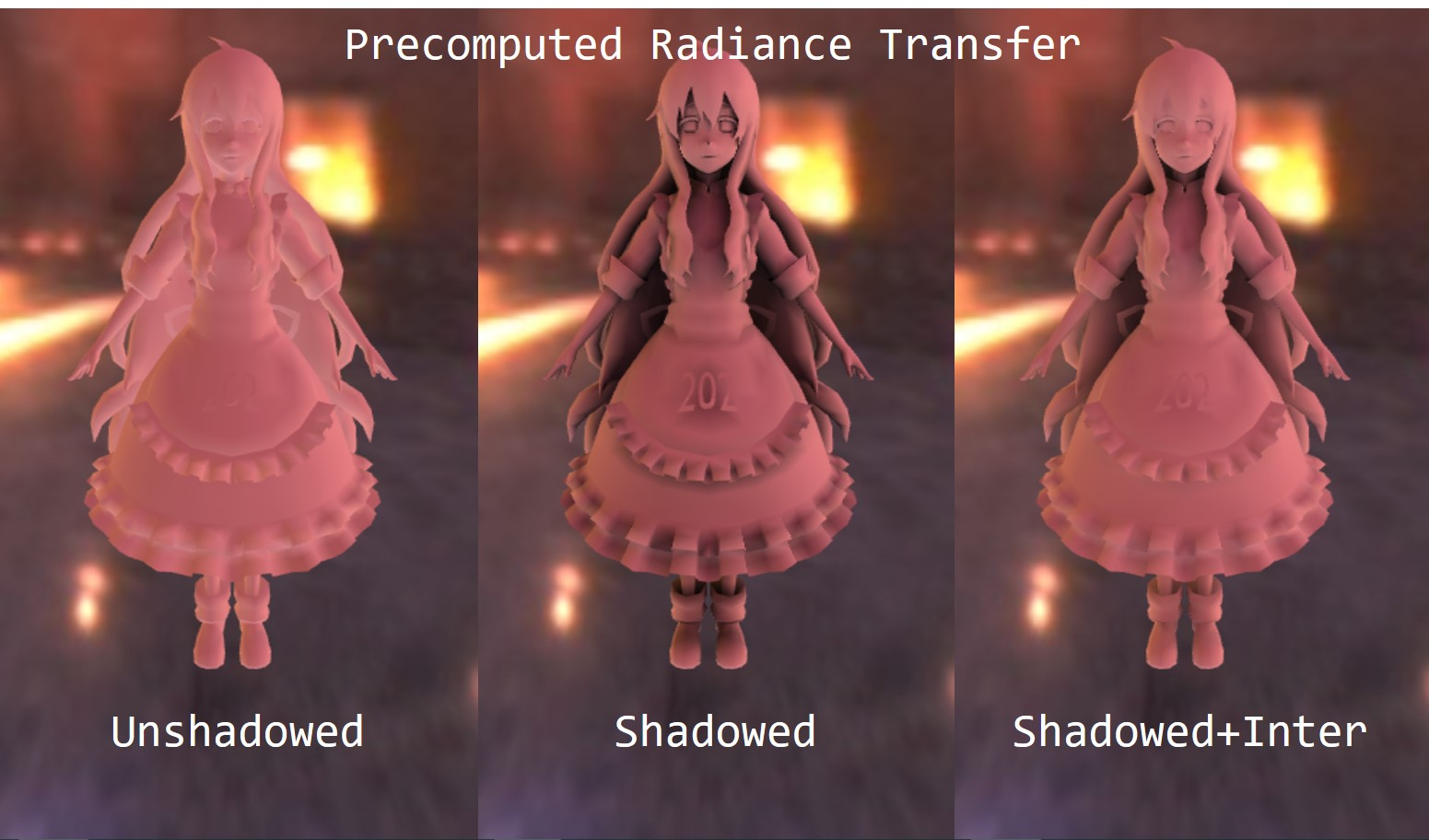## 一、前言

环境光照处理着色点与周围环境的光影交互，对增强物体与周围环境的融入程度有着至关重要的作用。在实时渲染领域，环境光特指由预先生成的环境贴图提供的光照信息（即天空盒），贴图的每个纹素都可以看成是一个光源（注：这些光源我们当成其在无穷远处），因此其覆盖的方向是三维空间的所有方向。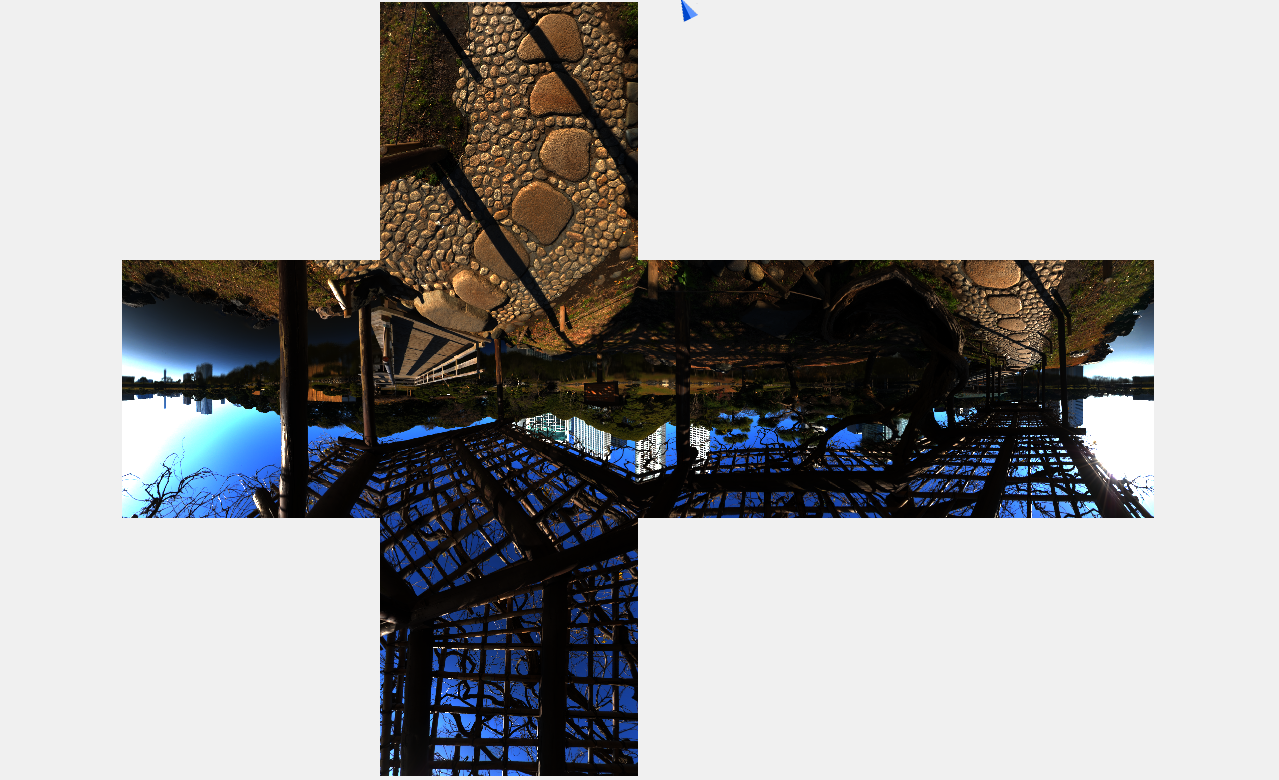理论上，准确求解环境光照需要计算如下的半球积分渲染方程：

其中，我们不考虑可见性遮挡因素，因此上式是去掉了$V(p,\omega_i)$项的。$L_i(p,\omega_i)$项通过用$\omega_i$对环境贴图进行采样获得。公式$(1)$的求解可以采用蒙特卡洛积分的方法结合重要性采样来进行求解，但这是离线渲染的做法，虽然理论上能够收敛到无偏的结果，但其耗费的算力代价和采样数量对于实时渲染来说根本不可接受！

由此衍生了一些快速的近似求解算法，例如IBL和PRT。这些算法的核心本质，就是预计算，通过某种方法把积分的求解放在预计算阶段，并将结果存储下来，在绘制阶段直接查表来实现快速的环境光渲染。

## 二、Imaged Based Lighting

IBL的本质就是Split Sum，把公式$(1)$的渲染方程进行拆解，达到变量降维的目的，实现低量存储、高速查表的性能。回顾上一节实时软阴影，我们提到实时渲染领域非常常用的一个近似公式：

该公式把两个函数乘积的积分近似为各自积分的乘积（即把乘积符号和积分符号做了个交换）。上次我们也提到，当函数$g(x)$满足以下两个条件时，公式$(2)$的近似是相对比较准确的：

• 当$g(x)$在积分域$\Omega$内的实际贡献（support）很小，即$g(x)$在$\Omega$内绝大部分取值为零，例如一个脉冲函数，仅在$x=0$不为零，其他地方均为零（即狄拉克函数）；
• 当$g(x)$在积分域$\Omega$内变化不大，可以理解为低频函数。

在实时渲染领域，有三种常用的BRDF材质：specular、diffuse和glossy，其中glossy是介于specular和diffuse之间的一种材质。三者的区别在于反射波瓣（lobe）的分布。如下图1所示，glossy的反射波瓣通常比较狭长（而specular则直接是一个方向了，其BRDF函数为狄拉克函数），diffuse反射波瓣在半球方向均匀分布。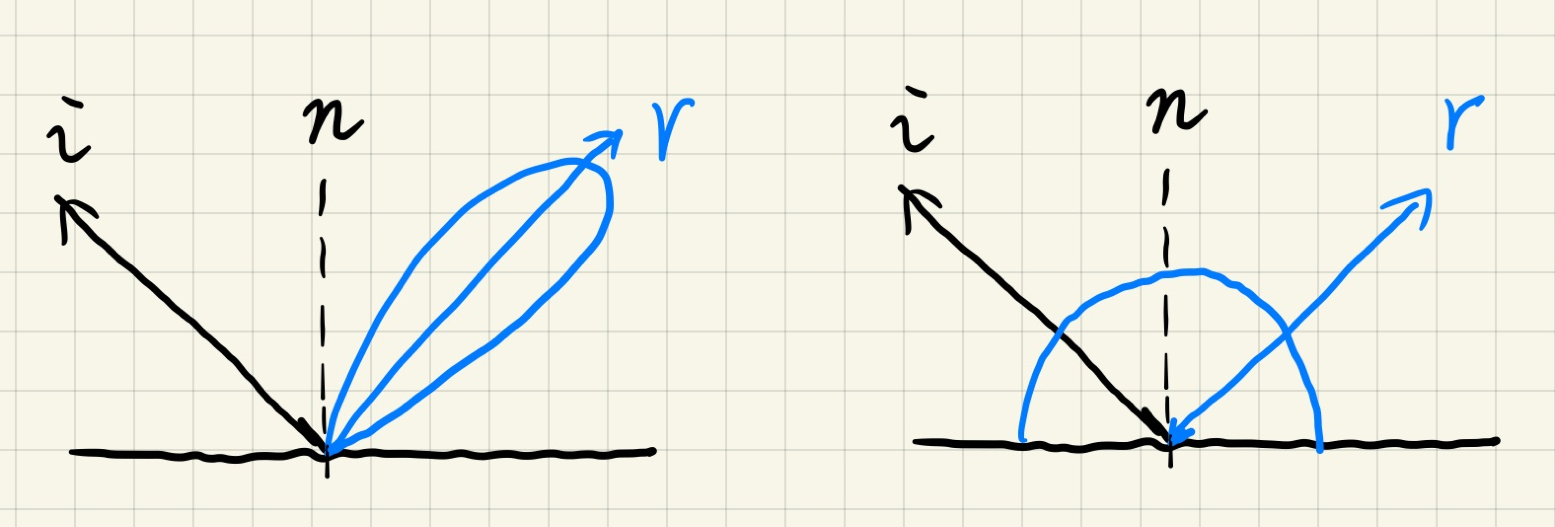因此，渲染方程$(1)$中的BRDF函数$f_r$在通常情况下，要么实际贡献范围很小（例如glossy），要么实际变化不大（例如diffuse）。由此可以利用公式$(2)$的近似模式来将渲染方程$(1)$拆解开来：

其中的积分区域$\Omega_{f_r}$为材质BRDF波瓣分布的区域。例如diffuse的话，那么就是整个半球；而对于glossy材质，其表面越粗糙，则$\Omega_{f_r}$越大。上式本质上可以看成为在$\Omega_{f_r}$区域上的对$L_i$做box filtering处理过程，不同的BRDF材质区别仅在于滤波区域$\Omega_{f_r}$的大小，材质越接近diffuse，那么其滤波范围越大，滤波得到的结果越模糊，反射的结果越模糊，反之则越光滑，这与我们的常识相符。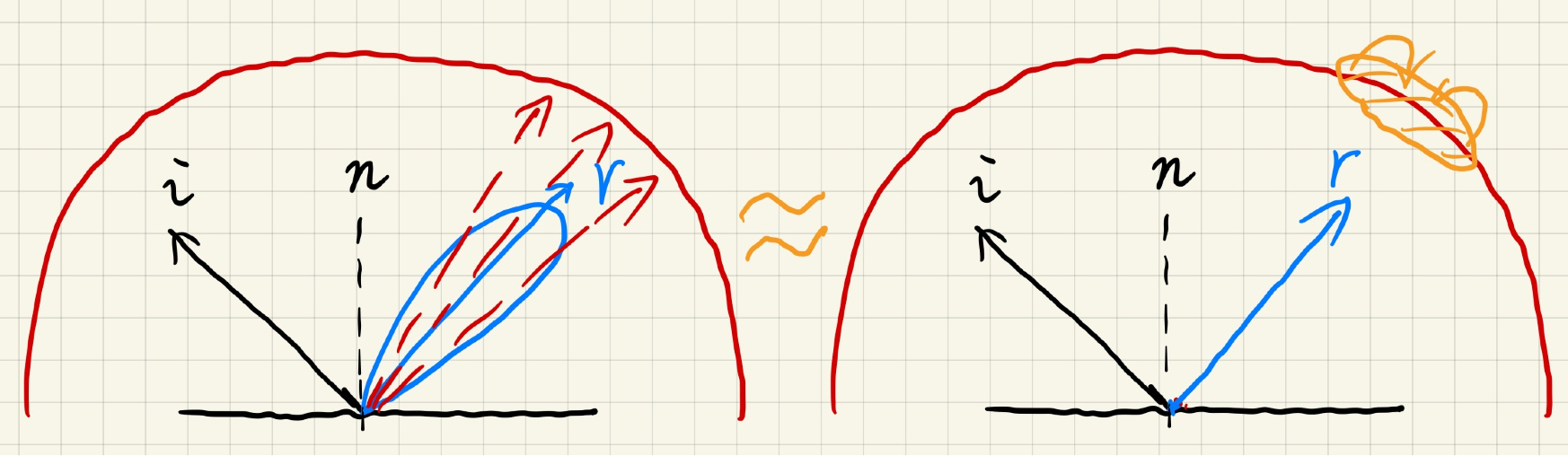brdf项的预计算核心公式为：

这个项的预计算不太好处理。在PBR材质流程管线，我们通常用如下的基于微表面的BRDF函数：

其中$F$、$G$和$D$分别是菲涅尔方程、几何遮蔽函数和法线分布函数。其中$h$是介于$\omega_i$和$\omega_o$之间的半角向量（half vector）。菲尼尔方程采用Schlick近似：

可以看到菲涅尔项依赖的参数有基础反射率$R_0$和$\omega_o\cdot h$。而$G$和$D$函数的可选项有很多，这里不罗列出来，但我们直到这两个函数依赖的参数为表面的粗糙度$\mu$。预计算的公式依赖的参数有三个，这说明我们需要三维的纹理来存储结果，为了降低依赖的维度，这里设法将一些变量提出积分符号外：

把公式$(5)$带入公式$(6)$，有：

然后把公式$(7)$得到的结果写成以下的两个部分：

这样我们就把$R_0$提出积分符号外面，其中下面的$f_r$是去掉了菲涅尔项的BRDF函数。剩下的两个积分项分别依赖于粗糙度$\mu$和$n\cdot \omega_o$，我们构建这样的一个查找表，横坐标$u$为$n\cdot \omega_o$，纵坐标$v$为$\mu$，每个$(n\cdot \omega_o,\mu)$对应的纹素的r通道和g通道分别存储公式$(8)$的左右两个积分项。如下图所示：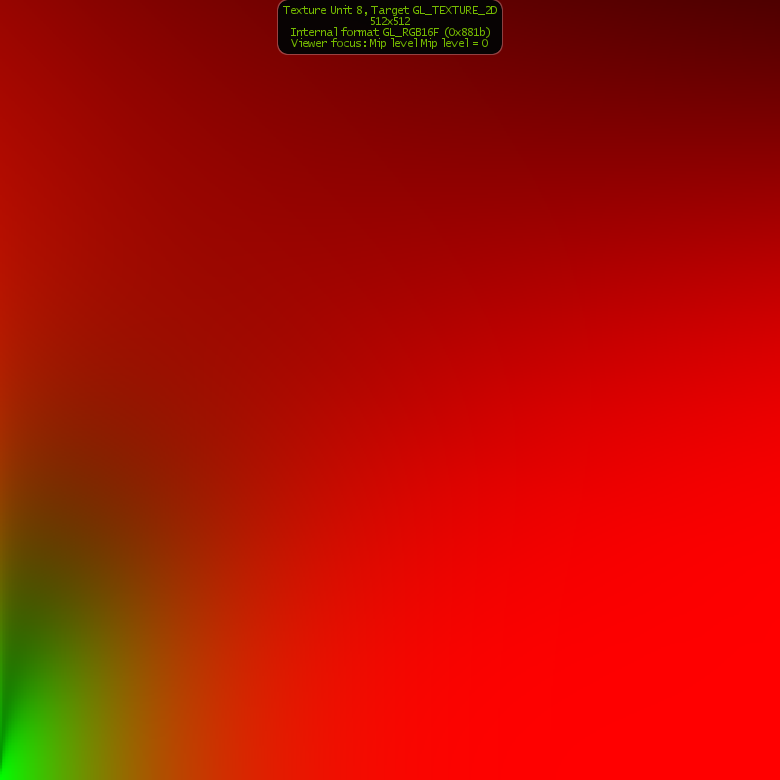关于IBL的实现详情见之前的博文实时渲染Real-time Rendering：Image Based Lighting，这里不再赘述。IBL缺点在于难以处理阴影遮挡问题，目前工业界主流的方法是为cubemap最亮的区域生成一个主要的阴影。

PRT涉及的数学内容较多，这里仅仅就个人理解做一个总结。PRT把渲染方程的辐射率项$L_i$和BRDF项展开成以球面谐波函数为基的系数权重，因此其预计算都是如何将函数投影到球面谐波函数表达的空间。

在高等数学、数学分析等课程中，我们都曾学习过函数的级数展开（例如泰勒展开）。对于给定的一个函数$f(x)$，我们可以对其进行级数展开，表示成一系列基函数的加权之和：

其中$B_i(x)$是第$i$个基函数，$c_i$是相应的权重。我们可以类比线性代数，把所有的基函数$B_i(x)$构成的空间看成是一个函数空间，$c_i$相应地就是在$B_i(x)$基底下的投影权重，所有的基底乘上相应的系数权重，线性组合成了原始函数$f(x)$。因此求解权重系数的过程我们称之为投影

以傅里叶变换为例。傅里叶变换本质上就是傅里叶级数展开过程中的投影，傅里叶级数用一系列不同频率、振幅的正余弦波的线性组合还原出原始函数$f(x)$。我们透过傅里叶权重可以更加方便地分析原始函数$f(x)$频率分布情况，进行傅里叶分析、滤波等操作。

卷积定理告诉我们，在空间域的卷积滤波等价于在频率域的乘积。因此，对于两个函数乘积的积分：

我们可以将上述的公式理解为低通滤波操作。滤波的结果取决于两个函数中比较低频的函数，这样较低频的函数把另一个较高频函数的高频信息过滤掉了！这个公式对于理解PRT非常重要。

球面谐波（Spherical Harmonics，简称SH）基函数是一组定义在球面上基函数，下图4展示了前4阶的SH基函数分布。第$i$阶SH基函数有$2i+1$个，前$i$阶一共有$(2i+1)^2$个SH基函数。与一维的傅里叶级数类似，越高阶的SH基函数其蕴含的频率信息越高。每个SH基函数由伴随勒让德多项式给出。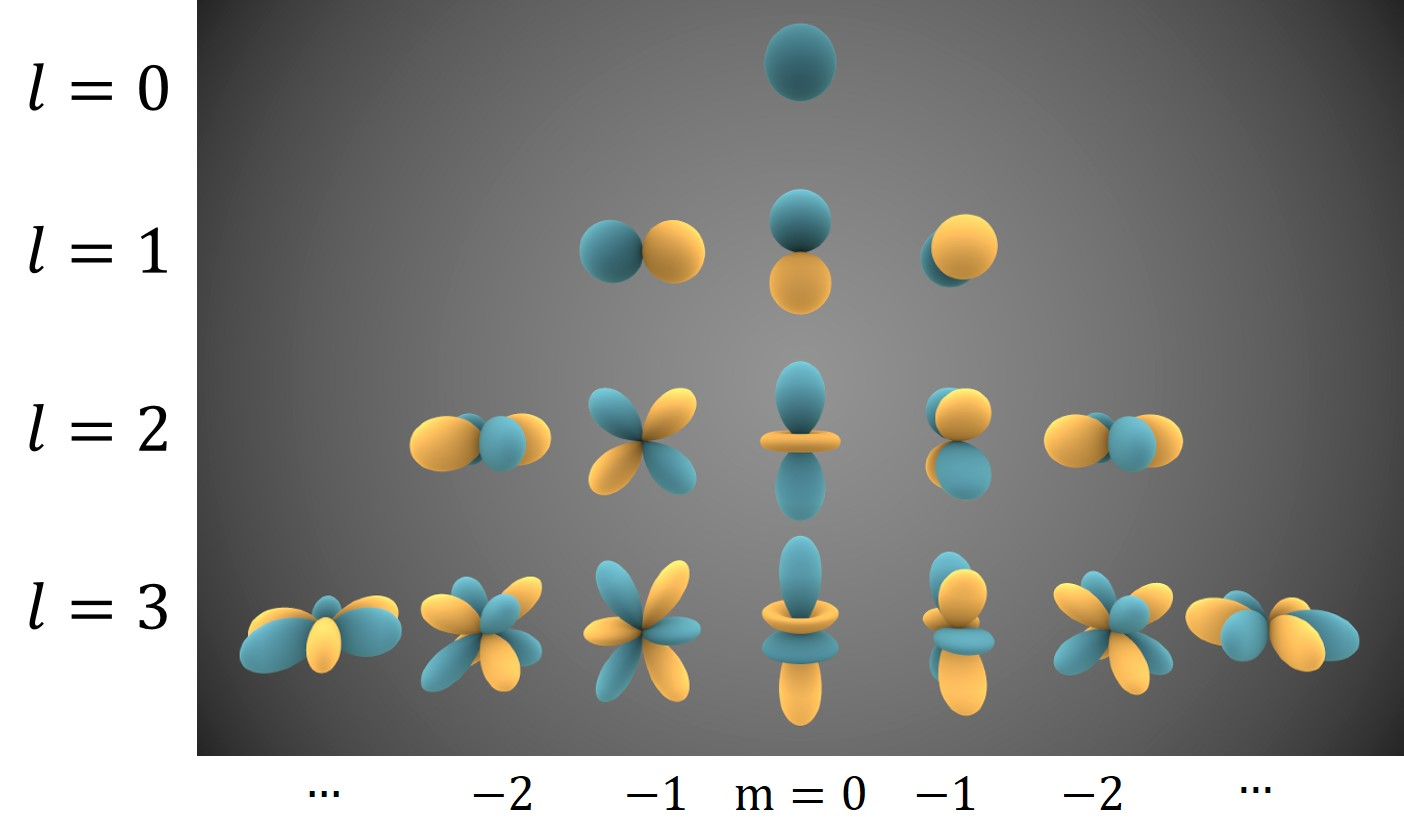这个球谐函数表给出了前几阶的SH基函数具体公式，这里不再赘述。这里特别提一点，SH基函数具备一个的良好性质：正交完备性，不同的基函数之间相互正交，即有：

而上式如果$i=j$，那么：

我们知道，三维的方向向量可以转换成用球面坐标系下的$(\theta,\phi)$来表示。因此任何一个关于三维方向$\omega$的函数$f(\omega)$都可以转换成球面函数，该函数在SH基函数$B_i(\omega)$下的权重计算（即投影）公式为：

投影之后，再用$c_i$权重带入公式$(9)$可以对原始函数$f(\omega)$进行复原，这个过程我们称之为重建。当然，由于级数有无穷多个，我们通常也就用前几阶的SH基函数进行重建，相应带来的影响就是去掉了后面SH基函数蕴含的高频信息（频率截断，即低通滤波），结果只是对原函数的近似。

我们把目光放回到渲染方程上面：

其中$\rho$是BRDF项，$V(i)$为可见性项。这个方程跟前面提到的形式不太一样，但本质不变，这里懒得改过来了。我们可以把积分里面的函数分成三类，分别是lighting项$L(i)$、visibility项$V(i)$和BRDF项$\rho(i,o)max(0,n\cdot i)$。如果我们直接暴力打表，为场景中的每个点，预计算存储上面的lighting项、visibility项和BRDF项，纹理分辨率为$64\times 64$，那么每个点的存储开销为$6\times 64\times 64$（之所以是$6$，是因为lighting项和BRDF项各占三个数，visibility项占一个数）。而且这仅仅是关于积分内的函数预存储，接下来还需要求解半球的积分方程！

PRT算法把前面公式$(12)$积分里面的函数分成两部分：$L_(i)$为lighting项，剩余的$V(i)\rho(i,o)max(0,n\cdot i)$被记为light transport项。先假定视角$o$固定不变，那么这两个项都是关于入射方向$i$的球面函数，都可以对其进行SH级数展开。

对lighting项的展开我们记为：

对light transport项的展开我们记为：

其中的$\omega_i$就是我们上面提到的入射方向$i$，$l_p$和$t_q$分别是相应的投影权重。将公式$(13)$和公式$(14)$带入渲染方程$(12)$，我们有如下的近似关系：

根据我们前面提到的正交完备性，可知当$p\neq q$时，积分结果为$0$；当$p=q$时，积分结果为$1$。故而公式$(15)$可以继续化简为:

最终的公式直接把积分去掉了！这就是PRT算法的神奇之处。公式$(16)$告诉我们，如果我们能够计算出$l_i$和$t_i$，那么渲染方程结果就是这两个乘积的和。而这所谓的乘积的和实际上就是两个向量的点乘，向量维数取决于采用了多少个SH基函数，如果采用前三阶SH基函数，那么一共有$9$个，$l$和$t$分别是一个$9$维向量，每一个维度是一个rgb向量，即$9\times 3$个数。注意，每个rgb通道各自采用上述的公式$(16)$独立计算（即$l_i\cdot t_i$并非两个rgb向量点乘，特别注意！！！），因此计算结果也是一个rgb向量。

总而言之，PRT算法的关键之处在于$l$和$t$的计算。$l$是lighting项的SH基函数投影，套用前面的投影公式$(11)$则有：

其中的积分区域$S$为球面所有方向。而$t$的计算类似，只是将light transport项代入投影公式即可：

这里的计算有一个比较棘手的地方。前面我们假设$\omega_o$固定不变，但如果$\omega_o$变了呢？对于diffuse的BRDF，其本身与$\omega_o$无关，因此$\omega_o$无论如何变化，在预计算阶段我们都可以直接忽略。但如果是glossy材质，$f_r$是会随着观察视角$\omega_o$的变化而变化的！为此，对于glossy材质的BRDF，我们写成如下形式：

即对于glossy材质，我们需要增加一个变量$\omega_o$进行打表。遍历$\omega_o$的离散取值，为每一个不同的$\omega_o$生成一个light transport向量$t(\omega_o)$，因此glossy材质的$t$是一个矩阵，矩阵规模为$m\times n$，其中$m$是SH基函数个数，$n$是$\omega_o$离散化个数。对于glossy材质，计算最终的光照辐射率时是$l$向量和$t$矩阵的矩阵向量乘。

总而言之，对于diffuse材质，我们用公式$(17)$和公式$(18)$预计算$l$向量和$t$向量；对于glossy材质，我们用公式$(17)$和公式$(19)$计算$l$向量和$t$矩阵。最后用公式$(16)$计算光照辐射率。注意预计算阶段，$l$向量只需计算一次，而$t$则需要遍历场景中的所有点（例如网格顶点），为每个点计算一个$t$，然后作为顶点的属性值一起传输到着色器中。这些积分公式的求解，有很多方法，例如最基本的黎曼和亦或者蒙特卡洛积分。

## 四、diffuse材质的PRT实现

这里以diffuse材质为例讲一下PRT的实现。PRT的实现分成两个步骤：预计算阶段和实时渲染阶段。对于diffuse材质，其BRDF为以下的lambertian公式：

其中，$\rho$为材质表面的反照率（diffuse颜色），这里我们假定其取值为$(1,1,1)$。因此，我们可以对前面的公式$(18)$进行简化，有：

把公式中的$V(\omega_i)$去掉就是不考虑场景自遮挡的情况。在这里我们只用前三阶SH基函数，那么一共有$9$个基函数，因此$p$取值为$0$到$8$。我们先来看lighting项的投影预计算，lighting项的投影公式为：

我们用黎曼和来对上述的积分公式进行近似：

在实现时，我们遍历cubemao的所有像素，每个像素构成一个方向$\omega_i$，套用上面的黎曼和公式计算。球谐函数直接调库，这方便的轮子没必要再自己去造。

对于light transport的预计算，也是对前面的公式$(21)$类似的计算过程。只不过需要为每个顶点都计算一个$t$向量，然后存储到文件中。可见性函数我们用光线与场景的求交来实现。

而如果要考虑多次弹射，那么可以通过迭代的方式计算$t$。在前一次迭代的基础上，从每个顶点发射一条射线，如果射线打中了另一个点，那么通过线性插值得到该点的前一次$t$，再乘以余弦项，最后累加。详情见下面代码（想偷懒了）。

得到了$l$和$t$，在渲染时我们通过存储的文件将这些数据加载进来，然后传到着色器中。Vertex着色器代码如下（重点关注LLT），这里实现的是Per-Vertex shading。

Fragment着色器代码如下所示：

实现的效果比较见下图。## Reference

$$ Sloan P P, Kautz J, Snyder J. Precomputed radiance transfer for real-time rendering in dynamic, low-frequency lighting environments[C]//Proceedings of the 29th annual conference on Computer graphics and interactive techniques. 2002: 527-536.

$$ GAMES202: 高质量实时渲染